## Introduction

Dynamical wavefunction collapse models1,2 provide a unified description of quantum dynamics encompassing both unitary evolution and state reduction. Typically the standard Schrödinger dynamics are extended so that the state behaves stochastically and in such a way that certain superposition states are unstable. The most prominent collapse model is the continuous spontaneous localization (CSL) model3,4 in which a superposition of quasi-localized matter states will collapse at a rate which increases with the mass of the object. This results in the rapid collapse of macroscopically distinguishable superposition states whilst micro states are little affected.

Since the CSL model involves a modification of the Schrödinger equation, it makes predictions which are in conflict with standard quantum theory. It is therefore possible to experimentally test CSL. A direct way to do this is to try to observe quantum interference for objects of increasing mass. Detailed studies show that there should be a characteristic loss of fringe visibility as the mass increases beyond a sufficient size (see Refs 5, 6, 7). Another observable effect of CSL is a random diffusive motion of particles. An isolated object will undergo a random walk induced by the collapse mechanism. As shown in Ref. 8 this can dominate over environmental effects at sufficiently low temperature and pressure.

Here we consider a situation with two non-interacting particles. We will examine how the particles behave as a result of the CSL dynamics and show that there is a potentially measurable effect whereby the diffusions undergone by each particle are correlated. A key feature of the CSL model is that the localization mechanism acts on the total smeared mass density state rather than individually on each particle. This suggests that for two nearby particles, the diffusive behaviour caused by the localization mechanism will be correlated. This should be apparent in the joint spatial probability distribution. In what follows we shall solve the two-particle CSL master equation to determine the behaviour precisely.

Our proposed experiment is shown schematically in Fig. 1. A pair of nanoparticles are trapped side by side using laser light. The particles are simultaneously released and allowed a brief period of free fall. The positions of each of the particles is then measured by light (Rayleigh) scattering. The experiment does not involve matter wave interferometry and therefore avoids much technical complication. Indeed the scheme can be performed with existing technology.

We assume that the two particles are non-interacting and of equal mass. We also assume that the two particles are identical bosons although this is not crucial to our argument—the same conclusion holds if the particles are non-identical. We could also regard the particles as composite objects made up of many constituent particles satisfying the CSL dynamics. This case is more complicated but it can be shown4 that the CSL dynamics can be applied to the composite object as a whole. Furthermore, any environmental particles involved in the CSL dynamics but not part of the system of interest can be traced away without effect.

There are two fixed parameters in the CSL model which are treated as fundamental constants. These are the localization rate λ and the localization length scale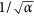. We consider a regime in which the localization length scale is large compared to the length scale defining the spatial extent of the system. The original estimates of these parameters by Ghirardi, Rimini and Weber (GRW)9 are λ = 10−16 s−1 and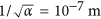. However, these values are not definitive. A recent study of the valid regions of the λ-α parameter space consistent with experiment puts no upper bound limit on the CSL length scale10.

The CSL master equation is given by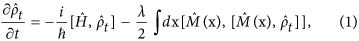with density operator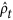and free Hamiltonian. The smeared mass density operator is given by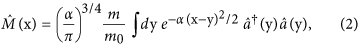where m0 is the nucleon mass (used to set the mass scale) and the field annihilation and creation operators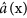and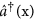satisfy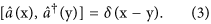The two-particle state is represented bywhere 1 and 2 label the two particles and ψ is a symmetric wavefunction. In this representation improper position eigenstates are given by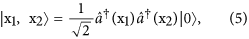and the two-particle density matrix is represented in coordinate space as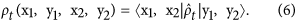The coordinate space representation of Eq. (1) for the two-particle state in the limit of large localization length is found to bewhere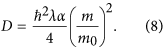Here, the limit of large localization length specifically means that ρt(x1, y1, x2, y2) is only significant whenever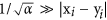for i = 1, 2 and(see Refs 11, 12).

Equation (7) applies in three dimensional space. In what follows we are concerned only with the behaviour in one dimension. We choose this to be the horizontal dimension of Fig. 1. We can trace over the coordinates of the other spatial dimensions in ρt resulting in a reduced one dimensional density matrix. It is straightforward to show that this satisfies a master equation with the same form as Eq. (7).

We now present a solution of Eq. (7) (further details of the techniques used can be found in Ref. 12). The solution is represented in terms of a density matrix propagator J (see Refs 13, 14) as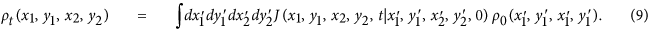We find that for Eq. (7) (in one dimension), J is given by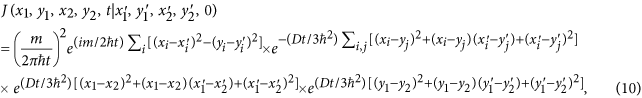where i, j = 1, 2.

We consider an initial wave function of the form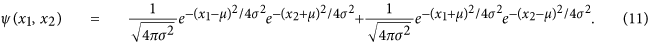This represents the particles residing in adjacent harmonic traps of width σ and spaced by a distance 2μ  σ. The initial density matrix is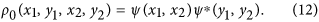We suppose that the particles are simultaneously released from the traps and allowed to diffuse freely. However, they remain sufficiently separated that there is negligible conventional interaction between them. Using Eqs (9 and 10) we have calculated the diagonal part of the density matrix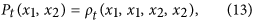following a time period t after their release. This represents the joint probability distribution for the subsequently measured positions of the two particles. The result is simplest when expressed in terms of the variables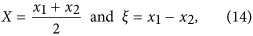where we find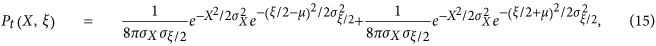with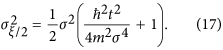The distribution consists of two peaks, one about X = 0, ξ/2 = μ and another about X = 0, ξ/2 = −μ. Note that we have ignored a possible interference term between the two peaks in Eq. (15) since we assume that they do not disperse enough to overlap. The feature that we are interested in is the shape of these peaks and in particular their rate of dispersion in the two directions X and ξ/2. The X and ξ spreads of each of the peaks are defined by σX and σξ/2 respectively. We see that the CSL parameter D contributes to σX whilst σξ/2 is unaffected. Note that by setting D = 0 (standard quantum mechanics) in Eqs (16 and 17) we find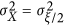. This reflects the fact that the two particles are behaving independently and their distributions are uncorrelated.

The physical reason for the difference between σX and σξ/2 when D ≠ 0 is that the diffusive shifts in position of the two particles caused by the collapse mechanism will be positively correlated if the particles are closer together than the localization length scale. The system as a whole diffuses but ξ = x2 − x1 is only affected by standard quantum dispersion.

Our result is clearly dependent on the fact that the localization length scale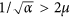, but in this limit the spreads in X and ξ/2 do not depend on the separation 2 μ. For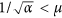the particles would behave independently under CSL. A null observation would therefore put a constraint on α.

To observe the effect we must distinguish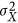and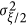. We can estimate these variances by repeatedly measuring the displacements of two particles simultaneously dropped from nearby traps (see Fig. 1). We assume that the traps are such that σ = 10 nm and the particles are released for t = 0.25 s corresponding to a drop of 30 cm. We further assume that position measurements have an error of σerr = 10 nm which is normally distributed and independent of xi. If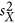is the unbiased estimate ofbased on n sets of position measurements, then the variance inis given by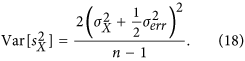To be able to clearly observe the effect of CSL we demand thatshould be at least 10 times smaller than the difference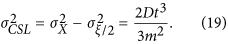Taking the mass of the particles to be 109 amu such that ħ2t2/4m2σ4 1, this results in the following constraint on n: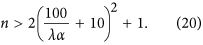This is shown in Fig. 2(a).

Now we consider the effects of an environment. We suppose that the particles are confined within a vacuum chamber and consider the constraints placed on temperature and pressure by the condition that the collapse effects should be dominant. We consider environmental noise contributions both from radiation and molecular collisions.

It is shown in Ref. 15 that the dominant contribution to the thermal diffusion by radiation is due to recoil from emission of photons. There it is also shown that the variance in displacement of a bulk sphere of radius R and density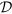due to the emission of radiation is given (in SI units) by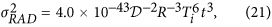where Ti is the internal temperature of the bulk object. By demanding that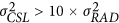we can constrain the internal temperature of the particles. Using= 103 kgm−3 and R = 10−7 m, we find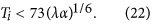The maximum internal temperature is shown in Fig. 2(b).

The mean time between molecule-sphere collisions in the impact regime is given in seconds by8where P is the pressure given in picoTorr, Te is the external temperature and T0 = 300 K is room temperature. We make the conservative assumption that τc should be 10 times greater than the total experiment time n × t. Taking Te/T0 1 this results in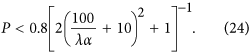The maximum ambient pressure in the vacuum chamber is shown in Fig. 2(c).

We conclude that an experiment can be performed to test for λα as low as 1 m−2s−1. This requires n = 24000; Ti = 73 K and P = 3.3 × 10 − 17 Torr. We assume a separation between traps of at least 1 mm (to avoid dispersion forces/gravity effects) so that the correlation effect should be present for. The accessible region of λ-α parameter space is shown in Fig. 3. Also shown is the region of parameter space currently ruled out by diffraction experiments involving particles of mass 105 amu16.